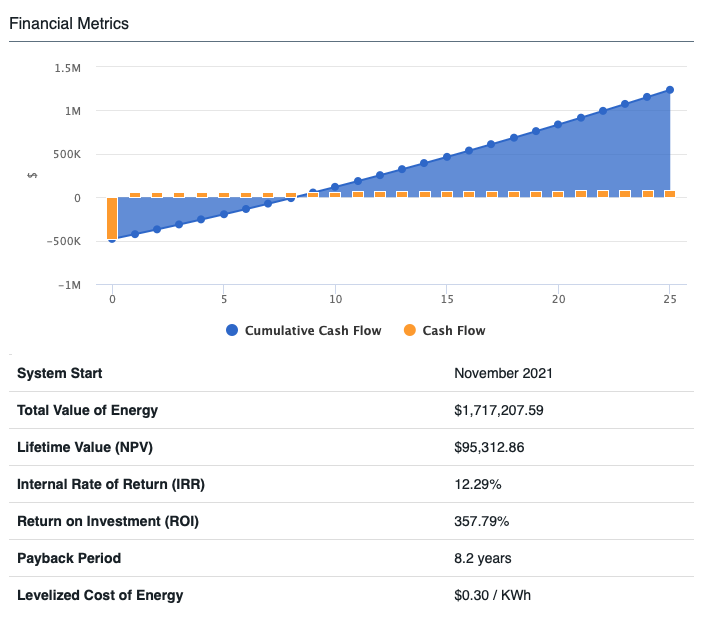# Explaining Financial Metrics like NPV, IRR, and ROI

When looking into the profitability of a project, investors rely on Financial Metrics like NPV, IRR, ROI, and LCOE. But, what do these metrics really mean?

### NPV (Net Present Value)

NPV represents the difference between the present value of cash inflows and cash outflows over a period of time. NPV is widely used for budgeting capital and allows investors to see if a project is profitable. When calculating NPV, we are looking to find today’s value of future payments as a general rule; projects with a negative NPV should be avoided.

NPV relies on the Discount Rate, which is the rate used to discount future cash flows in discounted cash flow. It also expresses the time value of money and can distinguish whether an investment project is financially viable or not.

### IRR (Internal Rate of Return)

The internal rate of return (IRR) is the expected compound annual rate of return that will be earned on a project or investment. It is a metric used in financial analysis to estimate the profitability of potential investments. It is widely used for decisions concerning how to use cash during budget planning.

One drawback of the IRR is its limited use with modeling financing options that require little to no initial investment out of pocket. For example, the IRR will return an infinitely high value if the project has no or low initial investment needed. So in cases where there is no down payment or a low one, the calculation will not depict a reliable metric of the investment's profitability.

### Payback Period

The Payback Period calculates how long it will take for the original investment to be recuperated. It is also described as the length of time an investment takes to reach its break-even point. It is important to remember that the payback period calculation is limited to the amount of time required to earn back initial investment costs. So if a loan with a low down payment is being analyzed, it is possible that the overall calculation won't represent a reliable picture of the investment since little to no upfront investment was used.

### ROI (Return on Investment)

The ROI is a financial metric widely used to measure the probability of gaining a return from an investment. The ROI represents a ratio that compares the gain or loss from an investment compared to its cost. It is calculated by subtracting the initial cost of the investment from the final salvage value of the investment (which represents the net return) and then dividing the net return by the cost of the investment and multiplying it by 100.

### LCOE (Levelized Cost of Energy)

The LCOE is a metric that measures the costs over the entire project lifetime divided by total energy production over that time period. Factors like the initial expenditure, financing costs (discount rate), electricity cost, operating expenses, and production will impact the LCOE.

#### How are these metrics included in a Proposal in HelioScope?

When creating a Proposal in our Financials Beta, after you select a Financial Configuration, we will automatically calculate the Financial metrics of your Project.